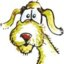MathematicsOpenStudy (anonymous):

Find the surface area of a right pyramid, if its height is 8 cm and its base is a rectangle with sides of length 4 cm and 12 cm. Assume the apex is centered over the base. Round to the nearest tenth......OpenStudy (anonymous):

formulaOpenStudy (anonymous):Directrix (directrix):OpenStudy (anonymous):

oh yes I was waiting for you :)Directrix (directrix):Directrix (directrix):

I was waiting to see if the other person were finished. I think I know what went wrong. Look at the new diagram.OpenStudy (anonymous):

okDirectrix (directrix):

Look at Tri ACD. Area of Tri ACD We have to get the height of the triangle by using the height of the solid. (AH)^2 + (HG)^2 = (AG)^2 8^2 + (HG)^2 = (AG)^2 8^2 + 2^2 = (AG)^2 64 + 4 = (AG)^2 68 = (AG)^2 8.2 = AGDirectrix (directrix):

Agree?OpenStudy (anonymous):

agreedDirectrix (directrix):

Look at triangle ACD in the improved diagram.Directrix (directrix):

Area of Tri ACD = 1/2 (12)(8.2) A = 6(8.2) A = 49.2 Area of Tri ABE also = 49.2 Sum of the areas of these two congruent triangles = 98.4. Check that before we move to the other triangles. Last time, I think I messed up by having the wrong sides labeled.OpenStudy (anonymous):

okayDirectrix (directrix):

Having a better diagram helps me. I should have drawn it better the first time. No worries. Live and learn.Directrix (directrix):

Look at Triangle ABCDirectrix (directrix):

Tri ABC To get its area, we will have to use the height of the solid )which is not the same as the altitude of triangle ABC to get the height of triangle ABC. Look inside the pyramid. 8^2 + (HF)^2 = (AF)^2 8^2 + 6^2 = (AF)^2 64 + 36 = (AF)^2 100 = (AF)^2 10 = AF Agree?OpenStudy (anonymous):

agreedDirectrix (directrix):

Area Tri ABC = (1/2) bh A = (1/2) (BC) * 10 A = (1/2) *4*10 A = 20 There are 2 of these triangles with the same area. Tri AED also has area 20. Total of the two is 40. Agree?OpenStudy (anonymous):

Okay I see.Directrix (directrix):

Alrighty, we are in the home stretch - we've been there before. :) But, this is the way learning goes. So, when you do your Geometry problems, always begin with detailed skeetch with all the given on it. Learn from my errors.Directrix (directrix):

The bottom of the pyramid is a 12 by 4 = 48 square units of area rectangle. So, the surface area of the pyramid is 48 + 98.4 + 40 = 186.4 cubic units. See if you agree.OpenStudy (anonymous):

I see, got it.Directrix (directrix):

Remember the words about always having a good diagram. And, if you ever feel you can't do math, remember that you CAN do math. There's nobody born who is good in math. It's working and sticking with it. I kinda like the adventure.OpenStudy (anonymous):

Thank You so much!Directrix (directrix):

You are welcome. We'll crank out another one of these problems soon. Suggestion. Start with this same problem from scratch and see if you can work it out for yourself. If you get lost, you can always look at this solution to see where to pick up. That is the way I studied mathematics. Practice ups performance.OpenStudy (anonymous):

Okay, that is fine. I will take a leave for now its late. Thank you again.

Latest Questionshayleed: in 800, charlemagne accepted the pope's offer to be crowned holy roman emperor. this act had the practical effect of a.
2 hours ago 0 Replies 0 MedalsGucchi: Object E has a mass of 4,800 kilograms. Object F has a mass of 600 kilograms. The weight of Object F will be ________ times the weight of Object E if both o
5 hours ago 5 Replies 1 Medalprettygirl09: i dont know wat this is but look closly its agirl starring at ze moon..... but te
1 hour ago 5 Replies 1 MedalGucchi: Read the list of substances below: Rubber, Steel, Glass, Iron Which substances ar
5 hours ago 7 Replies 1 MedalGucchi: In a chemical reaction, 36 grams of hydrochloric acid reacts with 40 grams of sodium hydroxide to produce sodium chloride and water.
5 hours ago 5 Replies 1 MedalGucchi: Which state of matter has particles that slide past each other while remaining in
5 hours ago 5 Replies 1 MedalGucchi: Which of the following is true about the mass of an object? It decreases if weight increases.
5 hours ago 6 Replies 1 MedalGucchi: A group of students is investigating how the addition of salt impacts the floatation of an egg in water.
5 hours ago 5 Replies 1 Medalhayleed: Which of the following was an effect of the Crusades on Jews? A. Jewish villages also became targets of the Crusaders.
5 hours ago 0 Replies 0 MedalsGucchi: An electrician cuts a 10-meter-long copper wire having a density 8.9 g/cm3 into 10 equal parts.
5 hours ago 5 Replies 1 Medal